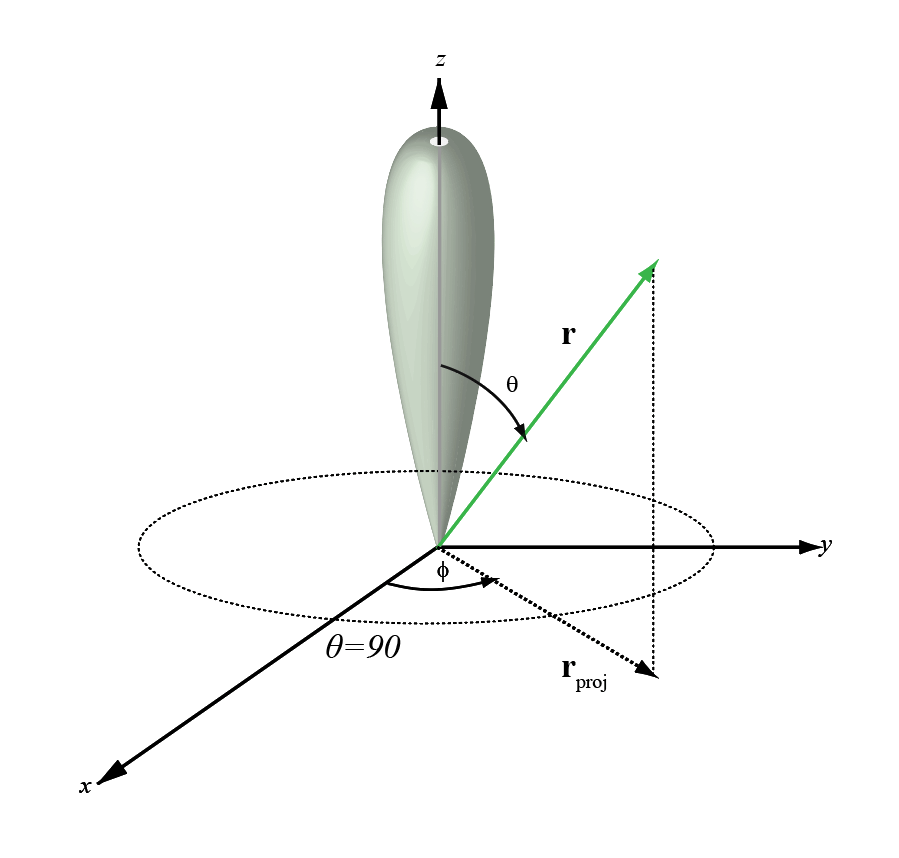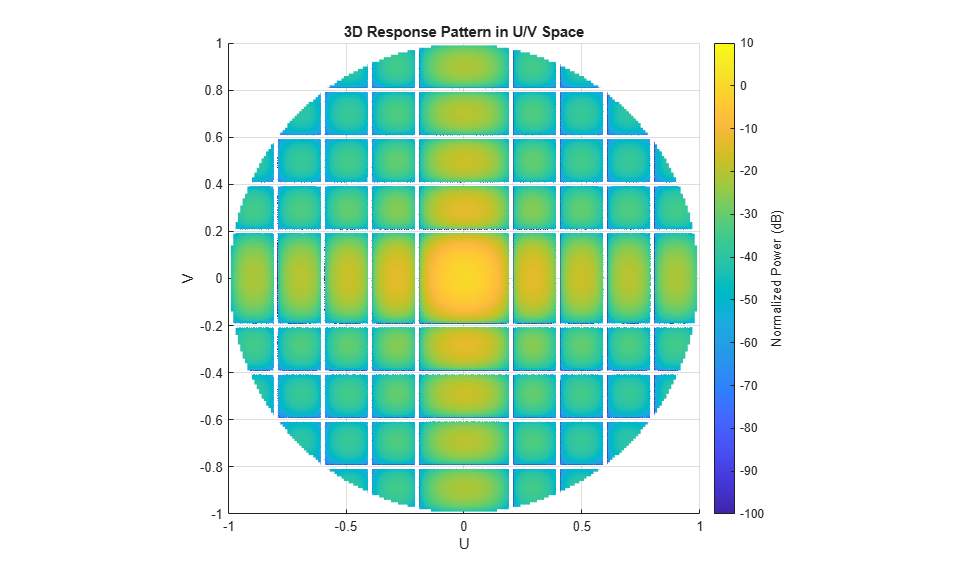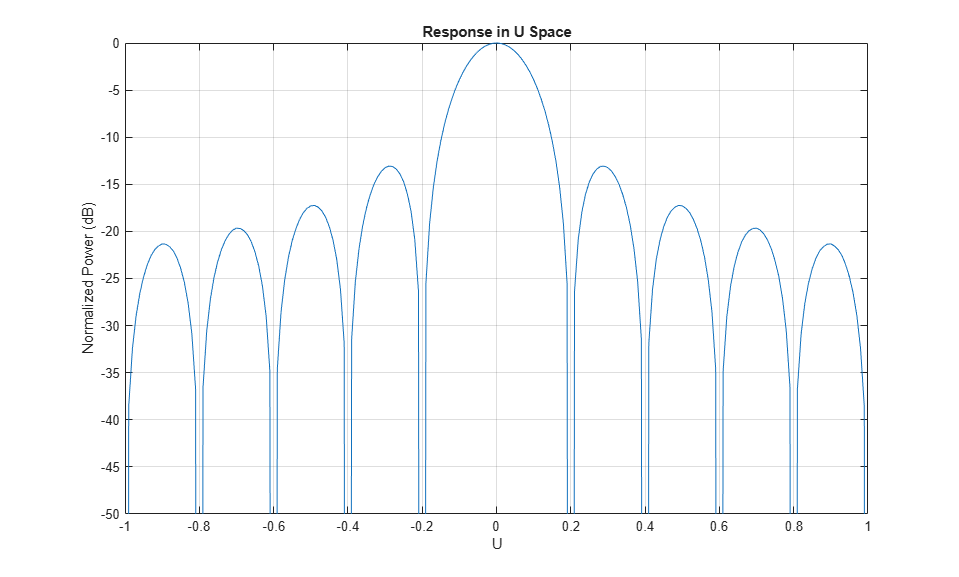Documentation

## Antenna Array Analysis with Custom Radiation Pattern

This example shows how to create an antenna array with a custom antenna radiation pattern and then how to analyze the array's response pattern. Such a pattern can be obtained either from measurements or from simulations.

Depending on the application, practical phased antenna arrays sometimes use specially designed antenna elements whose radiation pattern cannot be represented by a closed-form equation. Even when the element pattern is well understood, as is the case with a dipole antenna, the mutual coupling among the elements can significantly change the individual element pattern when the element is put into an array. This makes the closed-form pattern less accurate. Therefore, for high fidelity pattern analysis, you often need to use a custom radiation pattern obtained from measurements or simulations.

There is no standard convention for the coordinate system used to specify the radiation pattern, so the result from one simulation package often cannot not be directly used in another software package. For example, in Phased Array System Toolbox™ (PST), the radiation pattern is expressed using azimuth (az) and elevation (el) angles, as depicted in Figure 1. It is assumed that the main beam of the antenna points towardazimuth andelevation, that is, the x-axis. The value of az lies betweenandand the value of el lies betweenand. See Spherical Coordinates.Figure 1: Spherical coordinate system convention used in Phased Array System Toolbox™.

A frequently-used full-wave modeling tool for simulating antenna radiation patterns is HFSS™. In this tool, individual elements are modeled as if they were part of an infinite array. The simulated radiation pattern is represented as an M-by-3 matrix where the first column represents the azimith angle, the second column represents the elevation angle, and the third column represents the radiation pattern in dB. The coordinate system and the definitions ofandused in HFSS is shown in Figure 2. In this convention, the main beam of the antenna points along the z-axis which is usually points vertically. The HFSS coordinate system is rotated so that the main beams are parallel in the PST and HFSS conventions. The value ofis betweenandand the value ofis betweenand.Figure 2: Spherical coordinate system convention used in HFSS.Figure 3: Rotated spherical coordinate system convention used in HFSS.

Note that that HFSS coordinate system is not exactly the same as thecoordinate system used in Phased Array System Toolbox™. In HFSS, the beam mainlobe points along the z-axis and the plane orthogonal to the beam is formed from the x- and y axes. In PST, the beam mainlobe points along the x-axis and the plane orthogonal to the beam is formed from the y- and z axes. However, the definitions ofandwith respect to the main beamlobe and orthogonal plane are exactly the same as in PST. Therefore, to use a custom pattern expressed in the-convention, transform the pattern into the az-el convention using some of the angle transformation tools contained in PST. Here is one possible approach:

1. Define the azimuth and elevation angles where the radiation pattern is to be evaluated.

2. Convert the az-el angle pairs to their corresponding-pairs.

3. Interpolate the original radiation pattern at these computed-pairs.

For example, a cardioid-shaped antenna pattern is simulated in the-convention and is saved in a `.csv` file. This pattern needs to be converted to az-el convention before the pattern can be placed in an array. The helper function `helperPatternImport` reads the `.csv` file and reformats its contents into a two-dimensional matrix inand.

```[pattern_phitheta,phi,theta] = helperPatternImport; ```

The `phitheta2azelpat` function converts the pattern from-coordinates to az-el coordinates.

```[pattern_azel,az,el] = phitheta2azelpat(pattern_phitheta,phi,theta); ```

The transformed pattern can now be used to form a custom antenna element. Assume that this antenna operates between 1 and 1.25 GHz.

```freqVector = [1 1.25].*1e9; % Frequency range for element pattern antenna = phased.CustomAntennaElement('FrequencyVector',freqVector, ... 'AzimuthAngles',az,... 'ElevationAngles',el,... 'MagnitudePattern',pattern_azel,... 'PhasePattern',zeros(size(pattern_azel))); ```

To verify that the pattern has been correctly transformed, plot the response of the custom antenna element. Notice that the main beam points toazimuth andelevation.

```fmax = freqVector(end); pattern(antenna,fmax,'Type','powerdb') view(-150,20) ```### Construct Antenna Array

Consider a 100-element antenna array whose elements reside on a 10-by-10 rectangular grid, as shown in Figure 3. To ensure that no grating lobes appear, elements are spaced at one-half wavelength at the highest operating frequency. This rectangular array can be created using the following commands.Figure 3: A 10-by-10 URA.

```c = physconst('LightSpeed'); lambda = c/fmax; array = phased.URA('Element',antenna,'Size',10,'ElementSpacing',lambda/2) ```
```array = phased.URA with properties: Element: [1x1 phased.CustomAntennaElement] Size: [10 10] ElementSpacing: [0.1199 0.1199] Lattice: 'Rectangular' ArrayNormal: 'x' Taper: 1 ```

The total radiation pattern of the resulting antenna array is plotted below in u-v space. The pattern is a combination of both the element pattern and the array factor.

```pattern(array,fmax,'PropagationSpeed',c,'Type','powerdb',... 'CoordinateSystem','UV'); ```One can also easily examine the u-cut of the pattern as shown below.

```pattern(array,fmax,-1:0.01:1,0,'PropagationSpeed',c, ... 'CoordinateSystem','UV','Type','powerdb') axis([-1 1 -50 0]); ```### Steer the Beam in Azimuth

This section illustrates the idea of phase steering an array. An advantage of phased arrays over a single antenna element is that the main beam can be electronically steered to a given direction. Steering is accomplished by adjusting the weights assigned to each element. The set of weights is also called the steering vector. Each weight is a complex number whose magnitude controls the sidelobe characteristics of the array and whose phase steers the beam.

The example scans the main beam of the array fromazimuth toazimuth, with the elevation angle fixed atduring the scan.

```helperPatternScan(array) ``````clear helperPatternScan ```

### Plot Radar Vertical Coverage Diagram

When a radar is deployed in the field, its radiation pattern is modified by the surrounding environment. For example, reflections from the earth may enforce or attenuate the signal arriving at the target via the direct path. In addition, the refraction from the ionosphere can also introduce another path from the top. The resulting pattern in the elevation direction is often quite complicated and a radar engineer often needs to do a rough estimate of the vertical coverage during the system design stage. This section shows how to estimate the radar vertical diagram, also referred to as Blake chart, if the array is deployed at a height of 20 meters and covers a free space range of 100 km.

Derive the elevation pattern.

```el_ang = -90:90; arrayresp = phased.ArrayResponse('SensorArray',array, ... 'PropagationSpeed',c); el_pat = abs(arrayresp(fmax,el_ang)); % elevation pattern ```

```freespace_rng = 100; % in km ant_height = 20; % in m radarvcd(fmax,freespace_rng,ant_height,... 'HeightUnit','m','RangeUnit','km',... 'AntennaPattern',el_pat/max(el_pat),'PatternAngles',el_ang.'); ```The resulting diagram contains a curve in space along which the return signal level is a constant. It clearly shows that the main beam is severely modified by the reflection. For example, at a range of 100 km, the return from a target at an elevation angle ofis much smaller compared to a target at the same range at a nearby elevation angle. The curve also show that for certain angles, a target can be detected at as far as 200 km. This is the case when the reflected signal and the direct path signal are in phase, thus resulting in an enhanced return.

### Summary

This example shows how to construct and analyze an antenna array using a custom antenna pattern. The pattern can be generated using full-wave modeling simulation software with the-convention. The pattern can then be converted to az-el convention. The resulting array is used to generate a vertical coverage diagram and is also scanned fromtoin the azimuth direction to illustrate the phase steering concept.